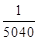# NCERT Solutions class-11 Maths Miscellaneous## myCBSEguide App

CBSE, NCERT, JEE Main, NEET-UG, NDA, Exam Papers, Question Bank, NCERT Solutions, Exemplars, Revision Notes, Free Videos, MCQ Tests & more.

Miscellaneous Exercise

1. A box contains 10 red marbles, 20 blue marbles and 30 green marbles. 5 marbles are drawn from the box, what is the probability that:

(i) all will be blue?

(ii) at least one will be green?

Ans. Total marks = 10 + 20 + 30 = 60

We have to draw 5 marbles out of 60 marbles in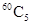ways.

(i) Probability that all will be blue =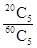(ii) Probability that at least one will be green =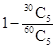2. 4 cards are drawn from a well-shuffled deck of 52 cards. What is the probability of obtaining 3 diamonds and one spade?

Ans. From a pack of 52 cards, 4 cards can be drawn in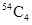ways.

There are 13 cards of diamond and 13 cards of spades.

Therefore, 3 cards of diamond out of 13 cards of diamond can be drawn in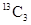ways and 1 card of spade out of 13 cards of spades can be drawn in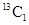ways.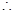Probability of obtaining 3 diamond and 1 spade card =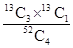3. A die has two faces each with number 1, three faces each with number 2 and one face with number 3. If die is rolled once, determine:

(i) P(2)

(ii) P(1 or 3)

(iii) P(not 3)

Ans. Total number of faces in a die = 6

Number of faces with 1 = 2, Number of faces with number 2 = 3 and Number of faces with number 3 = 1P(1) =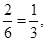P(2) =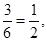P(3) =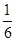(i) P(2) =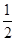(ii) P(1 or 3) = P(1) + P(3)

=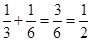(iii) P(not 3) = 1 – P(3)

=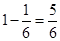4. In a certain lottery 10,000 tickets are sold and ten equal prizes are awarded. What is the probability of not getting a prize if you buy (a) one ticket (b) two tickets (c) 10 tickets.

Ans. Total number of tickets = 10,000 and Number of prize bearing tickets = 10

(a) Let A be the event that one ticket is prize bearing ticket.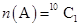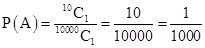and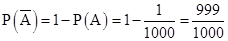(b) Let B be the event that two tickets are prize bearing tickets.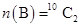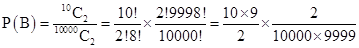=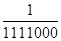And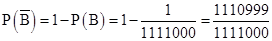(c) Let C be the event that ten tickets are not prize bearing tickets.Number of non-prize bearing tickets = 10000 – 10 = 9990P(C) =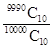5. Out of 100 students, two sections of 40 and 60 are formed. If you and your friend are among the 100 students, what is the probability that:

(a) you both enter the same section?

(b) you both enter the different sections?

Ans. Total favorable outcomes in which both the students are in same section of 40 students =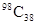Also total favorable outcomes in which both the students are in same section of 60 students =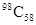P(both students are in same section) =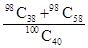=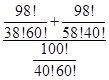=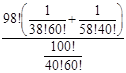=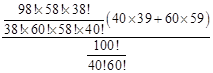=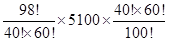=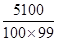=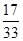And P(both students are in different section) =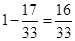6. Three letters are written to three persons and an envelope is addressed to each of them, the letters are inserted into the envelopes at random so that each envelope contains exactly one letter. Find the probability that at least one letter is in its proper envelope.

Ans. Number of ways in which three letters put into three envelopes = 3! = 6

Number of ways in which one letter put into correct envelopes =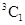=3

Number of ways in which two letters put into correct envelopes = 1

Number of ways in which at least one letter put into correct envelope = 3 + 1 = 4Probability that at least one letter put into correct envelope =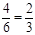7. A and B are two events such that P(A) = 0.54, P(B) = 0.69 and P(A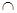B) = 0.35. Find:

(i) P(A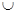B)

(ii) P(A’B’)

(iii) P(AB’)

(iv) P(BA’)

Ans. Given: P(A) = 0.54, P(B)

= 0.69 and P(AB) = 0.35

(i)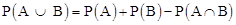= 0.54 + 0.69 – 0.35 = 0.88

(ii)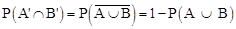= 1 – 0.88 = 0.12

(iii)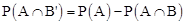= 0.54 – 0.35 = 0.19

(iv)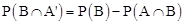= 0.69 – 0.35 = 0.34

8. From the employees of a company, 5 persons are selected to represents them in the managing committee of the company’s particulars of five persons are as follows: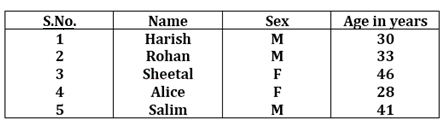A person is selected at random from this group to act as a spokesperson. What is the probability that the spokesperson will be either male or over 35 years?

Ans. Total number of persons = 5

One spokesperson is selected out o f5 persons in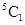= 5 ways.

Let A be the event that person is male and B be the event that person is over 35 years.

There are 3 male and one person can be selected inways.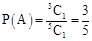There are 2 persons who are over 35 years therefore one person can be selected in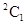ways.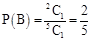There is 1 person who is male and over 35 years.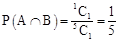And P(either male or over 35 years) =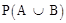=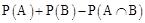=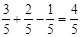9. If 4-digit numbers greater than 5000 are randomly formed from the digits 0, 1, 3, 5 and 7 what is the probability of forming a number divisible by 5 when (i) the digits are repeated (ii) the repetition of digits is not allowed?

Ans. (i) When digits are repeated: In a 4-digit number greater than 5000, thousands place

can be filled up by either 5 or 7.

Therefore, thousands place can be filled in 2 ways and remaining three places can be filled in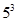= 125 ways.Total number of exhaustive cases =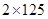= 250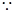A number is divisible by 5 if the unit place is either 0 or 5.Thousands place are fixed in 4 ways.

And the hundreds and tens place are to be filled in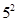= 25 waysRequired probability =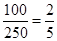(ii) When digits are not repeated: In a 4-digit number greater than 5000, thousands place can be filled up by either 5 or 7.

If the thousands place is filled by 5 then the hundreds, tens and unit place may be filled in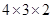= 24 ways.

Similarly, if the thousands place is filled by 7 then the hundreds, tens and unit place may be filled in= 24 ways.

A number greater than 5000 and divisible by 5 when unit place is either 0 or 5 and thousands place is either 5 or 7.

The tens and hundreds place can be filled in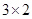= 6 ways.

Number of favorable cases =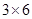= 18Required probability =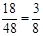10. The number lock of a suitcase has 4 wheels, each labelled with ten digits i.e., from 0 to 9. The lock opens with a sequence of four digits with no repeats. What is the probability of a person getting the right sequence to open the suitcase?

Ans. Total digits from 0 to 9 = 10

Since the digits cannot be repeated, therefore, the first place may be filled in 10 ways, second place in 9 ways, third place in 8 ways and fourth place in 7 ways.Number of possible outcomes =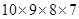= 5040

The lock of suitcase can be opened in 1 way only.Number of favorable cases = 1Required probability =